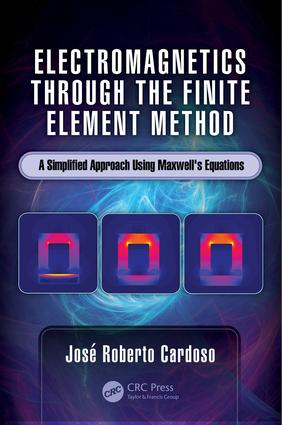# Electromagnetics through the Finite Element Method

## A Simplified Approach Using Maxwell's Equations, 1st Edition

CRC Press

198 pages | 16 Color Illus. | 109 B/W Illus.

##### Purchasing Options:\$ = USD
Hardback: 9781498783576
pub: 2016-10-14
SAVE ~\$31.00
\$155.00
\$124.00
x
eBook (VitalSource) : 9781315366777
pub: 2016-10-03
from \$28.98

FREE Standard Shipping!

### Description

Shelving Guide: Electrical Engineering

Since the 1980s more than 100 books on the finite element method have been published, making this numerical method the most popular. The features of the finite element method gained worldwide popularity due to its flexibility for simulating not only any kind of physical phenomenon described by a set of differential equations, but also for the possibility of simulating non-linearity and time-dependent studies. Although a number of high-quality books cover all subjects in engineering problems, none of them seem to make this method simpler and easier to understand.

This book was written with the goal of simplifying the mathematics of the finite element method for electromagnetic students and professionals relying on the finite element method for solving design problems. Filling a gap in existing literature that often uses complex mathematical formulas, Electromagnetics through the Finite Element Method presents a new mathematical approach based on only direct integration of Maxwell’s equation. This book makes an original, scholarly contribution to our current understanding of this important numerical method.

### Reviews

"There will be ‘purists’ that will see in this book an over-simplification of the subject. But engineers, including myself will like it precisely because of the simplifications afforded. In the end, for an engineer, the important thing is that ‘it works’ and that one understands how it works. I cannot find anything negative to say about it. I am glad that Prof. Cardoso finally decided to write the book."

—Nathan Ida, The University of Akron, Ohio, USA

"This is a novel approach to teaching electromagnetics through numerical methods. The fundamentals are sound and students need much less math sophistication compared to traditional methods. The approach I believe will motivate students and does provide insights not available with classical texts."

—Sheppard Salon, Rensselaer Polytechnic Institute, Troy, New York, USA

### Table of Contents

Steps for Finite Element Method. Introduction. Steps for Finite Element Method. Step 1: 2D versus 3D. Step 2: Definition of the Domain. Step 3: Selection of the Type of Element and Discretization. Step 4: Selection of the Interpolator Function. Step 5: Selection of the Type of Study. Step 6: Introduction of the Boundary Conditions. Step 7: Solution of the Equation System. Step 8: Exploration of Results—Postprocessing. Fundamentals of Electromagnetism. Sources of Electromagnetic Field. Volumetric Distribution of Electric Charges. Superficial Distribution of Electric Charges. Linear Distribution of Electric Charges. Discrete Electric Charges. Current Density Vector. Current Superficial Density Vector. Field Vectors. The Electric Field Vector. The Magnetic Field Vector. Displacement Vector. Magnetic Field Intensity Vector. The Electric Polarization Vector. The Magnetization Vector. Quantities Associated with Field Vectors. Voltage between Two Points. Magnetic Flux. Concatenated Magnetic Flux. Electromotive Force. History. Magnetomotive Force. Constitutive Relations. Maxwell’s Equations. Introduction. Maxwell’s First Equation. The Curl of a Vector Field. Maxwell’s Second Equation. The Displacement Current. Total Current Crossing a Surface. Maxwell’s Third Equation. Divergence of a Vector Field. Gauss’s or Divergence One’s Theorem. Maxwell’s Fourth Equation. The Continuity Equation. Where Are We?. A Little Bit of History. Stationary Regime. Quasi-Static Regime. Complex Representation of Sinusoidal Quantities. Magnetodynamics. Fast Time-Dependent Electromagnetic Fields. Boundary Conditions. Normal Components of B and H. Normal Components of D and E. Normal Components of J . Tangential Components of H. Tangential Components of E. Tangential Components of J. Electromagnetism Potentials. The Scalar Electric Potential—V. The Scalar Magnetic Potential—ψ. The Magnetic Potential Vector—A. The Electric Potential Vector. Potentials in Time-Dependent Fields. Finite Element Method in Static, Electric, and Magnetic Fields. Introduction. Steps in FEM Application. 2D Electrostatics. 2D Interpolator Function. Element’s Matrix in Electrostatics. 2D Electrokinetics: Stationary Currents Field. Magnetostatics. Assembling of the Global System of Equations. Introduction of the Boundary Conditions. Introduction of Dirichlet Conditions. Introduction of Neumann Conditions. Introduction of Special Boundary Conditions. Periodicity Conditions. Floating Boundary Condition. Nonlinear Magnetostatics. Magnetostatics with Permanent Magnets and Anisotropic Media. Finite Element Method for Time-Dependent Electromagnetic Fields. Introduction. Quasi-Static Electromagnetic Field. Alternating Current Steady-State Magnetodynamics. Numerical Example: Magnetodynamics. Calculus of the Matrix Element. Evaluation of Action Vectors. Assembly of Global Matrix and Introduction of Boundary Conditions. Evaluating the Eddy Current Induced on Element (1). Finite Element Method for Axisymmetric Geometries. Introduction. Electrostatics in Axisymmetric Geometry. Electric Charge Internal to Surface. Electrokinetics in Axisymmetric Geometry. Magnetostatics in Axisymmetric Geometry. Concatenated Current with the Polygonal Line. Magnetodynamics in Axisymmetric Geometry. Finite Element Method for High Frequency. Introduction. Special Features of Maxwell’s Equations. TM Mode; Hz = 0. TE Mode; Ez = 0. Three-Dimensional Finite Element Method. Introduction. Shape Functions for a Tetrahedron Element. 3D Electrostatics. 3D Interpolator Function. 3D Electrokinetics. Results Exploration. Introduction. Results Exploration. Conclusion. References. Index

### About the Author

Jose Roberto Cardoso was born in Marilia, SP Brazil, in 1949. He received his B.S., M.S. and Ph.D degree in electrical engineering from Escola Politecnica da USP, SP, Brazil, in 1974, 1979 and 1986, respectively. Since 1999, he has been a Professor with the Energy and Electrical Automation Department, Escola Politecnica da USP. He is the author of three books, more than 70 articles, and has advised more than 40 theses/dissertations. His research interests include numerical methods for electromagnetics, theoretical electromagnetics, electrical machines, electromechanical biomedical application, electrical railway research and engineering education. He is the former president of SBMAG – Brazilian Electromagnetics Society and former Dean of Escola Politecnica da USP. He is the founder of the LMAG – Electromagnetic Applied Laboratory. Prof. Cardoso was a recipient of the 2013 Emeritus Engineer of the Year in Sao Paulo.

### Subject Categories

##### BISAC Subject Codes/Headings:
TEC019000
TECHNOLOGY & ENGINEERING / Lasers & Photonics
TEC024000
TECHNOLOGY & ENGINEERING / Microwaves